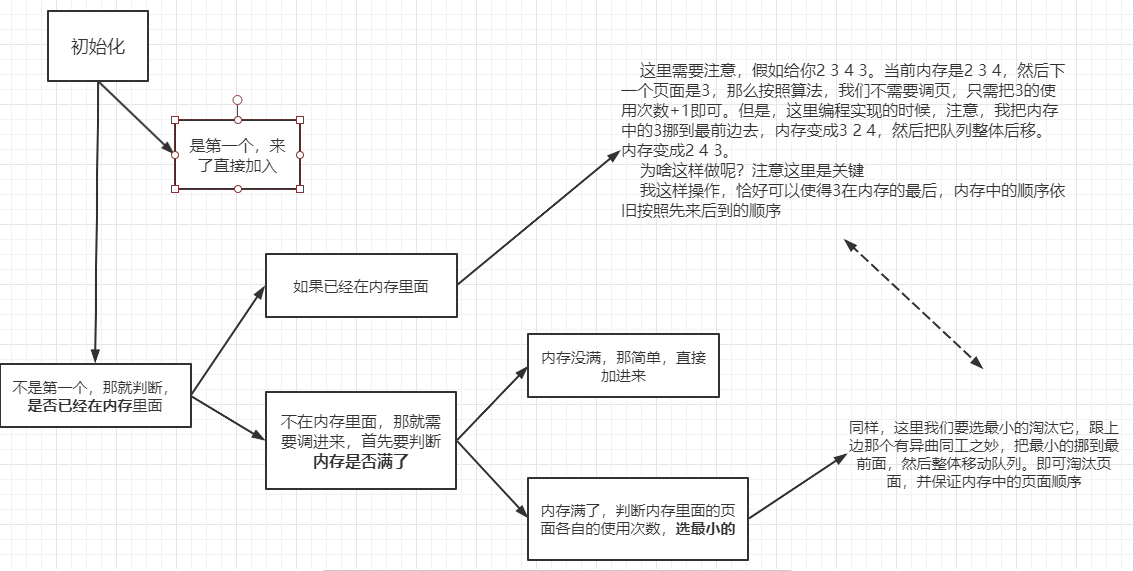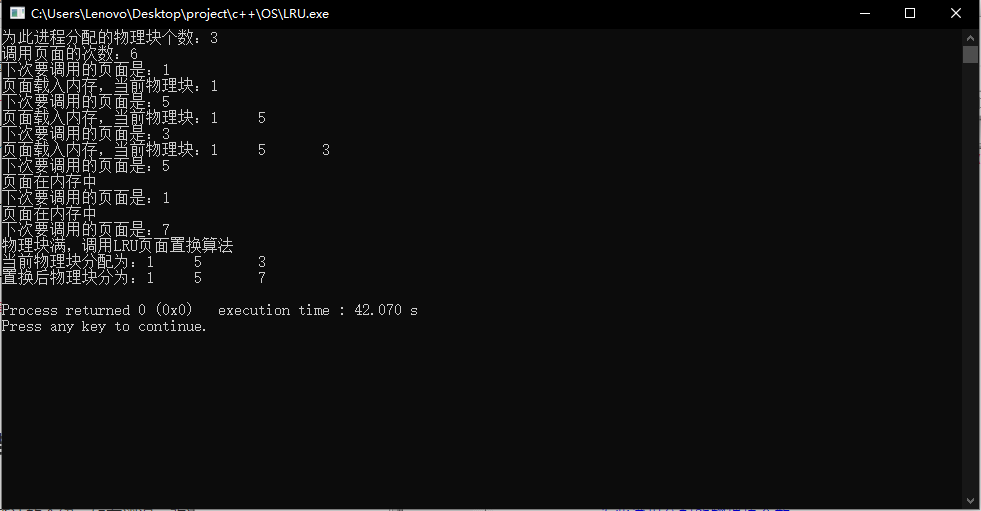• 使用LRU算法实现页面置换算法LRU算法基于一种假设，长期不使用的数据，在未来的使用性也不大。因此，当数据占用内存达到一定的阙值时，我们要移除最近最少使用的数据。LRU算法中，使用了一种有趣的数据结构，叫做...
• 页面置换算法LRU （1）建立相应的数据结构； （2）在屏幕上显示物理块的变化，可逐步展示或完整展示； （3）设计页面访问序列，可以展现你所设计的页面置换算法； （4）计算页面的缺页次数、缺页后的页面置换次数
• 页面置换算法LRU（模拟页面管理）  用高级语言编写一个页面置换算法LRU的模拟程序。  设置恰当的数据结构，有效存储数据。   动态输入存储区块数、作业调度序列。  输出分配结果、置换过程以及其它相关的...页面置换算法LRU
• LRU页面置换算法实现 一请求分页存储系统,进程P共有5页,访问串为3,2,1,0,3,2,4,3,2,1,0,4,试采用LRU算法和LFU算法分析,当分配页面数为3时,发生的缺页中断次数
• LRU页面置换算法，使用Java语言编写，使用策略模式，可以方便的换成其他页面置换算法！相当好！
• FIFO、OPT、LRU页面置换算法实验代码和截图操作系统
• 基于linux C模拟FIFI和LRU页面置换算法页面置换算法

#include

#include

#include

#include

using namespace std;

HANDLE hmove=CreateSemaphore(NULL,1,1,"yw_zh"); //同步信号量

int xl={7,0,1,2,0,3,0,4,2,3,0,3,2,1,2,0,1,7,0,1};

int i=0,d=32768;

int zy=0; //记录寄存器需要首位置1的物理块号

struct phyblk

{

unsigned int regit; //存放16位寄存器数值

int psave; //记录存放的页面

bool state;

phyblk(){regit=0;psave=0;state=0;}

};

phyblk pb; //3个物理块

int main()

{

cout<

<

return -1;

return -1;

system("pause");

}

{

int a=0,b=0,c=0,qy=0;

while(i<20)

{

Sleep(80);

WaitForSingleObject(hmove,INFINITE);

for(a=0;a<3;a++)

if(pb[a].psave==xl[i]&&pb[a].state==1) //判断页面是否已经在内存中

{

zy=a;

goto outcyc; //跳出for循环

}

for(a=0;a<3;a++)

{

if(pb[a].state==0) //寻找空物理块

{

pb[a].psave=xl[i];

zy=a;

pb[a].state=1;

goto outcyc;

}

if(pb[a].regit

{

d=pb[a].regit;

b=a;

}

}

zy=b;

cout<

pb[b].state=1;

pb[b].psave=xl[i];

qy++; //记录缺页数

d=32768;

outcyc:

i++;

}

cout<

return 0;

}

{

int a=0,b=0,c=0;

while(i<20)

{

Sleep(100);

for(a=0;a<3;a++)

pb[a].regit>>=1; //向右移1位

pb[zy].regit+=32768; //首位置1

ReleaseSemaphore(hmove,1,NULL);

}

}

展开全文• LRU的思想实现缺页中断以及页面置换。C语言实现，按照最近最久未被访问的思想去实现这个算法。输出每一次页面置换之后的过程以及最后的缺页中断次数与缺页中断率。LRU 操作系统
•代码
• 由于原代码测试中有些问题，因此我经过修改后上传，此代码VS2010下可直接使用，优化了原代码中当物理块数较大因此在前物理块数个页面中即有命中时的页面置换失常现象。可以实现LRU、OPT、FIFO算法打印置换情况并计算...
• （1）输入一个逻辑页面访问序列和随机产生逻辑页面访问序列，由四个线程同时完成每个算法； （2）能够设定驻留内存页面的个数、内存的存取时间、缺页中断的时间、快表...(7) 给出每种页面置换算法每个页面的存取时间；C#
• LRU页面置换算法C语言实现

千次阅读 2021-05-10 11:00:08
LRU 最近最少使用页面置换算法 思路： 代码： #include<stdio.h> #include<string.h> void Change(int P[],int x,int y){//将p数组中x位置的元素挪到y位置 //我们默认传参进来的 x大于 y 也...

思路：代码：

#include<stdio.h>
#include<string.h>

void Change(int P[],int x,int y){//将p数组中x位置的元素挪到y位置
//我们默认传参进来的 x大于 y  也就是默认往前挪  故不用判断x y大小关系了
int temp= P[x];
while(x!=y){  //依次后移
P[x]=P[x-1];
x--;
}
P[y]=temp;
}

int main(){
int PageNum;
int Count;
int Sum,MaxNum;
memset(Count,0,sizeof(Count));
scanf("%d",&Sum);//总数
scanf("%d",&MaxNum);//内存可存放页框数量
for(int i=1;i<=17;i++)  //初始化
scanf("%d",&PageNum[i]);
//页面调度
int LackPage=0;
int q=1,w=1;
int flag=0;
for(int i=1;i<=Sum;i++){
if(i==1){  //第一个必然要直接加入
Count[ PageNum[i] ]++;
w++; //此时为第一个 无需考虑内存已满情况
LackPage++;//缺页次数加1
}
else{
flag=0;
for(int j=q;j<w;j++){
if(PageNum[j]==PageNum[i]){//如果内存中已存在该页面
flag=j;//记录该页面位置
}
}
if(flag!=0){ //内存中已存在该页面，需要把该页面挪到第一个位置
//ps:
//若flag-q=0 说明已经是第一个位置了 就不用挪
//所以 小于0  需要挪
if( (flag-q) > 0){
Change(PageNum,flag,q);
}
q++;//挪完了，直接给他移出去
w++;//把新的加进来 （注意：这里不用判断内存满不满，因为根本没增加数量
Count[ PageNum[i] ]++; //该页面使用次数 +1
}
else{//否则就是内存中没有该页面
LackPage++;//先把缺页次数+1
//下面开始调页
if(flag==0 && (w-q) < MaxNum){//内存中没有该页面且内存未满
Count[ PageNum[w] ]++;//使用次数加1
w++; //直接加入内存
}
else{//内存中没有该页面且内存已经满了
//注意：这里才是这个算法的核心，我们要比较使用次数
flag=q;//flga用于记录使用次数最少的页面位置
//这里flag初试指向q是有意义的，不能乱改
//如果大家使用次数都一样，那么q刚好是先进来的那个
for(int j=q+1;j<w;j++){//比较次数
if(Count[ PageNum[j] ] < Count[ PageNum[flag] ] )
flag=j;//记录最小的
}
if(flag==q){//说明使用次数都一样，那就选最先进来的
q++;
w++;
Count[ PageNum[i] ]++; //该页面使用次数 +1
}
else{
Change(PageNum,flag,q);//将使用次数最少的挪到最前面
q++;//淘汰使用次数最少的
w++;//加入新的
Count[ PageNum[i] ]++; //该页面使用次数 +1
}
}
}
}
}
printf("一共执行了 %d 个页面\n",Sum);
printf("内存可以存放 %d 个页面\n",MaxNum);
printf("一共缺页 %d 次\n",LackPage);
printf("缺页率 %.3f \n",LackPage*1.0/Sum);
return 0;
}

展开全文• LRU页面置换算法C++代码

千次阅读 2019-08-17 00:55:09
LRU页面置换算法C++代码 常见的缓存算法： LRU：(least recently used) 最近最少使用，思想是如果一个数据刚被访问过，那么将来被访问的几率也更高 LFU：(least frequently used) 最少经常使用，思想是如果一个...

LRU页面置换算法C++代码

常见的缓存算法：

• LRU：(least recently used) 最近最少使用，思想是如果一个数据刚被访问过，那么将来被访问的几率也更高
• LFU：(least frequently used) 最少经常使用，思想是如果一个数据很久不被访问，那么将来被访问的几率也不大
• FIFO：(first in first out) 先进先出，思想是如果一个数据最先进入缓存，则应该最早淘汰掉

我们这里主要看一看LRU缓存的规则：

• 新数据插入到链接头部
• 每当数据被访问，则将数据移到链表头部
• 当链表满的时候，则将链表尾部的数据删除，在将新数据插入链表头部

LRU：cache应该具备的两个操作：

1. get（key）：如果key在hashmap中存在，则直接查找其在list链表中的位置，然后将其移动到链表头部再更新其hashmap的映射
2. set（key，value）：如果key在hashmap中不存在，并且页面还满了，则删除尾部节点，再插入，如果存在于hashmap中，则将其从list链表中移动到链表头部，再更新其hashmap的映射

我们现在自己手动实现一下：

我们这里LRU使用的是list + map来实现，采用list的原因是可以快速移动数据，而map用来保存节点的key，value的值，便于能快速根据key值找点其在list中对应的位置，所以value值保存的是list的迭代器类型

list + map的代码如下：

//查找时间复杂度 是map 红黑树 logn
#include <iostream>
#include <vector>
#include <list>
#include <map>
#include <hash_map>
using namespace std;

struct MyLRUnode
{
int key;//形成key_value结构，通过这个唯一的键 直接可以找到这个值
int value;
MyLRUnode(int a, int b):key(a), value(b){}
};

class LRUCache
{
public:
LRUCache(int size)
{
Lru_capacity = size;
}
int get(int key) //访问键为key的页面 找到 则将其重新插入到链表头
{
if(mymap.find(key) != mymap.end())//如果找到了
{
mylist.splice(mylist.begin(), mylist, mymap[key]);//则将mylist链表上找到的的iterator位置的元素重新链接到mylist.begin()位置上
mymap[key] = mylist.begin();//然后将map映射表中 键为key的页面映射位置重置一下
return mymap[key]->value;//最后返回将key对应的value返回
}
else//没找到 则返回-1
{
return -1;
}
}

void put(int key, int value)//访问key value页面是否存在，若存在则提前“并更新value值”，不存在则创建之后再提前
{
if(mymap.find(key) == mymap.end())//key值不在页中
{
if(mylist.size() == Lru_capacity)//不在页中并且页还满了
{
mymap.erase(mylist.back().key);//将list尾部节点对应的key和其页映射关系删除
mylist.pop_back();//将list尾部节点删除
}
MyLRUnode pnewnode(key, value);//创建新页
mylist.push_front(pnewnode);//插入页链表中
mymap[key] = mylist.begin();//key对应页面关系存入map
}
else//key值在页中
{
mymap[key]->value = value;//更新key对应的value值
mylist.splice(mylist.begin(), mylist, mymap[key]);//将mylist链表上找到的的iterator位置的元素重新链接到mylist.begin()位置上
mymap[key] = mylist.begin();//然后将map映射表中 键为key的页面映射位置重置一下
}
}

void show_list()
{
list<MyLRUnode>::iterator it = mylist.begin();
for(;it != mylist.end();)
{
cout << it->key << ":" << it->value <<endl;
it++;
}
cout << endl;
}
void show_map()
{
map<int, list<MyLRUnode>::iterator>::iterator it = mymap.begin();
while(it != mymap.end())
{
cout << it->first << ":" << (it->second)->value << endl;
it++;
}
cout << endl;
}
private:
int Lru_capacity;
list<MyLRUnode> mylist;
map<int, list<MyLRUnode>::iterator> mymap;//用来通过key键值 直接找到对应的双向链表节点
//hash_map<int, list<MyLRUnode>::iterator> myhash_map;
};

int main()
{
LRUCache lru(3);
lru.put(1,100);
lru.show_list();
lru.show_map();
lru.put(2,200);
lru.show_list();
lru.show_map();
lru.put(3,300);
lru.show_list();
lru.show_map();
lru.get(2);
lru.show_list();
lru.show_map();
lru.put(4,400);
lru.show_list();
lru.show_map();
return 0;
}

如果现在我们需要对其进行改进，map查找的时间复杂度还是有点大，是O(logn)，我们怎么对其进行修改，修改为时间复杂度为O(1)呢？

说到O(1)，我们第一时间想到的是哈希，所以我们可以使用hash_map将map替换掉即可，底层的hash_map会根据传入的key值，通过hash函数建立哈希表，以达到查找时间为O(1)

list + hash_map的代码如下：

//查找时间复杂度 是hash_map 映射表 查找时间复杂度 O(1)
#include <iostream>
#include <vector>
#include <list>
#include <map>
#include <hash_map>
using namespace std;

struct MyLRUnode
{
int key;//形成key_value结构，通过这个唯一的键 直接可以找到这个值
int value;
MyLRUnode(int a, int b):key(a), value(b){}
};

class LRUCache
{
public:
LRUCache(int size)
{
Lru_capacity = size;
}
int get(int key) //访问键为key的页面 找到 则将其重新插入到链表头
{
if(myhash_map.find(key) != myhash_map.end())//如果找到了
{
mylist.splice(mylist.begin(), mylist, myhash_map[key]);//则将mylist链表上找到的的iterator位置的元素重新链接到mylist.begin()位置上
myhash_map[key] = mylist.begin();//然后将map映射表中 键为key的页面映射位置重置一下
return myhash_map[key]->value;//最后返回将key对应的value返回
}
else//没找到 则返回-1
{
return -1;
}
}

void put(int key, int value)//访问key value页面是否存在，若存在则提前“并更新value值”，不存在则创建之后再提前
{
if(myhash_map.find(key) == myhash_map.end())//key值不在页中
{
if(mylist.size() == Lru_capacity)//不在页中并且页还满了
{
myhash_map.erase(mylist.back().key);//将list尾部节点对应的key和其页映射关系删除
mylist.pop_back();//将list尾部节点删除
}
MyLRUnode pnewnode(key, value);//创建新页
mylist.push_front(pnewnode);//插入页链表中
myhash_map[key] = mylist.begin();//key对应页面关系存入map
}
else//key值在页中
{
myhash_map[key]->value = value;//更新key对应的value值
mylist.splice(mylist.begin(), mylist, myhash_map[key]);//将mylist链表上找到的的iterator位置的元素重新链接到mylist.begin()位置上
myhash_map[key] = mylist.begin();//然后将map映射表中 键为key的页面映射位置重置一下
}
}

void show_list()
{
list<MyLRUnode>::iterator it = mylist.begin();
for(;it != mylist.end();)
{
cout << it->key << ":" << it->value <<endl;
it++;
}
cout << endl;
}
void show_map()
{
hash_map<int, list<MyLRUnode>::iterator>::iterator it = myhash_map.begin();
while(it != myhash_map.end())
{
cout << it->first << ":" << (it->second)->value << endl;
it++;
}
cout << endl;
}
private:
int Lru_capacity;
list<MyLRUnode> mylist;
//map<int, list<MyLRUnode>::iterator> mymap;//用来通过key键值 直接找到对应的双向链表节点
hash_map<int, list<MyLRUnode>::iterator> myhash_map;
};

int main()
{
LRUCache lru(3);
lru.put(1,100);
lru.show_list();
lru.show_map();
lru.put(2,200);
lru.show_list();
lru.show_map();
lru.put(3,300);
lru.show_list();
lru.show_map();
lru.get(2);
lru.show_list();
lru.show_map();
lru.put(4,400);
lru.show_list();
lru.show_map();
return 0;
}

展开全文• 操作系统LRU页面置换算法 C语言程序 数组实现 简单，清晰且实用，
• LRU页面置换算法模拟，vs2010，面向对象设计
• 文章目录LRU页面置换算法前言一、LRU算法介绍二、代码1.源程序2.运行结果 前言 LRU页面置换算法是虚拟存储器中使用的算法，通过选取最近最少使用的页面换出 一、LRU算法介绍 LRU（最近最少使用）页面置换算法是...

前言

LRU页面置换算法是虚拟存储器中使用的算法，通过选取最近最少使用的页面换出

一、LRU算法介绍

LRU（最近最少使用）页面置换算法是根据过去使用次数来预测将来的页面使用情况，具体过程如下：
当发生缺页而分配给进程的物理块耗尽时，需要将所需页面置换入内存，被换出的页面是最近最少被使用的页面。具体的实现方法为为每个内存中的页面配置一个移位寄存器，每过一段时间（如10ms）将其中的数字右移。当该页面被访问时，将最高位置一，这样，最近被访问的页面寄存器中的数字是最大的，需要换出页面时，只需选取数值最小的页面换出；另一种方法是为每个进程配置一个栈，页面进入内存时将其压入栈中，当访问内存中的页面时，将其重新压入栈顶，如此最近被访问的页面就在栈顶，需要置换页面时，只需将栈底的页面置换出即可。下面代码使用的是第一种方法。

二、代码

1.源程序

#include <iostream>
#include <vector>
using namespace std;

typedef struct
{
unsigned visit;
///int block = ;
int state;
int pageId;
}PageItem,*itemp;

itemp initItem(int pageId)
{
itemp aItemp = new PageItem;
aItemp->visit = 0b0;
aItemp->state = 0;
aItemp->pageId = pageId;
return aItemp;
}

void moveright(itemp items[],int num)
{
for(int i=0;i<num;i++)
items[i]->visit = (items[i]->visit)>>1;
}
void LRU(itemp items[],int num,int pageId,vector<int>& blocks)
{
int id = 0;
unsigned visit = 0xFFFFFFFF;
for(int i=0;i<num;i++)
{
if(visit>items[i]->visit && items[i]->state==1)
{
visit = items[i]->visit;
id = items[i]->pageId;
}
}
for(int i=0;i<num;i++)
{
if(items[i]->pageId==id)
{
items[i]->visit = 0b0;
items[i]->state = 0;
}
if(items[i]->pageId==pageId)
items[i]->state = 1;
}
for(vector<int>::iterator i=blocks.begin();i!=blocks.end();i++)
{
if(*i==id)
{
*i = pageId;
break;
}
}
}

int main()
{
int invoke = 0;
int blocknum = 0;
int next = 0;
int blockTaken = 0;
vector<int> blocks(0);
itemp items = {};
for(int i=0;i<8;i++)
items[i] = initItem(i);
///PageTable table(5,items);
cout << "为此进程分配的物理块个数：";
cin >> blocknum;
cout << "调用页面的次数：";
cin >> invoke;
for(int i=0;i<invoke;i++)
{
cout << "下次要调用的页面是：";
cin >> next;
bool in = false;
for(vector<int>::iterator i=blocks.begin();i!=blocks.end();i++)
{
if(next == *i)
{
in = true;
break;
}
}
if(in)
{
cout << "页面在内存中\n";
}
else if(blockTaken<blocknum)
{
//页面不在内存中并且物理块还未耗尽
blocks.push_back(next);
cout << "页面载入内存，当前物理块：";
for(vector<int>::iterator i=blocks.begin();i!=blocks.end();i++)
cout << *i << '\t';
cout << endl;
items[next]->state = 1;
blockTaken++;
}
else if(blockTaken>=blocknum)
{
cout << "物理块满，调用LRU页面置换算法\n";
cout << "当前物理块分配为：";
for(vector<int>::iterator i=blocks.begin();i!=blocks.end();i++)
cout << *i << '\t';
LRU(items,5,next,blocks); //物理块耗尽，使用LRU算法置换页面
cout << endl << "置换后物理块分为：";
for(vector<int>::iterator i=blocks.begin();i!=blocks.end();i++)
cout << *i << '\t';
cout << endl;
}

items[next]->visit+=0b10000000000000000000000000000000;
moveright(items,8);
}
return 0;
}

2.运行结果以上是我对LRU算法的理解，如有谬误，欢迎斧正。

展开全文• LRU页面置换算法

万次阅读 2019-06-09 19:45:59
LRU是Least Recently Used的缩写，即最近最少使用，常用于页面置换算法，是为虚拟页式存储管理服务的。 为了尽量减少与理想算法的差距，产生了各种精妙的算法，最少使用页面置换算法便是其中一个。LRU算法的提出，是...操作系统
• 该工程具体是在codeblock上面实现了操作系统课程上讲解的页面置换算法，包括先进先出（FIFO）、最佳置换算法（OPT）、最久最近未使用算法（LRU）。 具体实现功能有： 1、建立相应的数据结构 2、在屏幕上显示页面...
• LRU页面置换，也称为最近最少使用算法 每个进程运行时会被分配一定数量的帧，这是进程所占有的空间。 程序的代码一般是存于外存（一般理解为磁盘）中 执行代码的时候，只有当代码所在的页被操作系统从外存调入内存，...
• 这是一个自己完成软件工程的操作系统课程课程设计题目：此程序用于模拟虚拟磁盘页面置换算法，实现了FIFO页面置换算法和LRU页面置换算法，获得课程设计优秀的好成绩页面置换算法
• 使用栈实现LRU页面置换算法代码 LRU页面置换算法可以使用基于计数器，或者基于栈两种方式进行实现 基于计数器 若有不当之处，请您指出，您的指点是我的荣幸 代码实现 import java.util.Scanner; /** * LRU页面...
• 写这个算法，就是一步一步调试出来的，花点功夫，其实原理比较简单，我使用的是链表。 中间有一些循环控制变量和条件需要琢磨以下，其他还好。...第二种情况，如果实页满了，进行页面置换 第三种情况，此时刻的页c语言 操作系统
• 操作系统实验报告，用C++实现 最近最久未使用LRU,先来先服务页面置换算法FIFO页面置换算法
• i++){ if(element[i]==k){ cout该引用已经存在于页帧中，故无需进行页面置换\n"; flag=1;break; } } if(flag==0){ int mind=1e6+10,j=0; cout没有空闲帧且该引用不存在于当前的页帧中，开始进行页面置换\n"; for(int...os
• 该工程具体是在codeblock上面实现了操作系统课程上讲解的页面置换算法，包括先进先出（FIFO）、最佳置换算法（OPT）、最久最近未使用算法（LRU）。 具体实现功能有： 1、建立相应的数据结构 2、在屏幕上显示页面的...页面置换算法
• ### 什么是LRU页面置换算法 LRU(Least Recently Used)，是页面置换算法的一种，它的主要思想是当用于缓存页面的地址块已满时，选择最久未使用的页面删除，然后用将新请求的页面加入缓存。 例如：请求为 {1,2, 0, 3...
• 模拟先进先出FIFO，最佳置换OPI和最近最久未使用LRU页面置换算法的工作过程 报告册和源程序
• LRU页面置换算法模拟-最近最久未使用置换算法LRU页面置换算法模拟-最近最久未使用置换算法|课程设计|计算机数据库课程设计一、设计目的1、用C语言实现最近最久未使用(LRU)置换算法。2、了解内存分页管理策略3、掌握...
•算法设计...